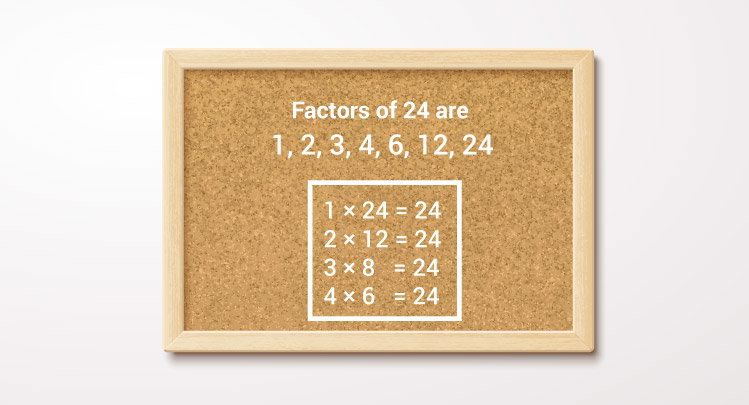# C Program to Display Factors of a Number

In this example, you will learn to find all the factors of an integer entered by the user.To understand this example, you should have the knowledge of the following C programming topics:

This program takes a positive integer from the user and displays all the positive factors of that number.

## Factors of a Positive Integer

``````#include <stdio.h>
int main() {
int num, i;
printf("Enter a positive integer: ");
scanf("%d", &num);
printf("Factors of %d are: ", num);
for (i = 1; i <= num; ++i) {
if (num % i == 0) {
printf("%d ", i);
}
}
return 0;
}
``````

Output

```Enter a positive integer: 60
Factors of 60 are: 1 2 3 4 5 6 10 12 15 20 30 60
```

In the program, a positive integer entered by the user is stored in num.

The `for` loop is iterated until `i <= num` is false.

In each iteration, whether num is exactly divisible by i is checked. It is the condition for i to be a factor of num.

``````if (num % i == 0) {
printf("%d ", i);
}
``````

Then the value of i is incremented by 1.